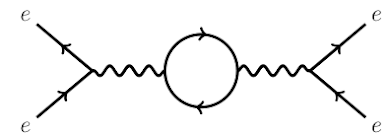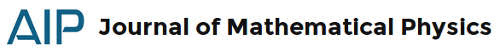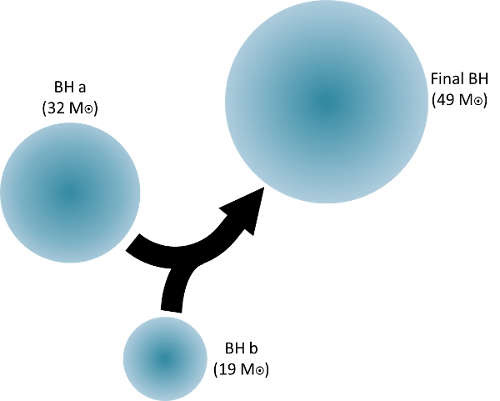### Wigner's theorem

The Wigner’s theorem was formulated and demonstrated for the first time by Eugene Paul Wigner on Gruppentheorie und ihre Anwendung auf die Quantenmechanik der Atomspektrum(1). It states that for each symmetry transformation in Hilbert’s space there exists a unitary or anti-unitary operator, uniquely determined less than a phase factor.
For symmetry transformation, we intend a space transformation that preserved the characteristics of a given physical system. Asymmetry transformation implies also a change of reference system.
InvariantsInvariants play a key role in physics, being the quantities that, in any reference system, are unchanged. With the advent of quantum physics, their importance increased, particularly in the formulation of a relativistic quantum field theory. One of the most important tools in the study of invariants is the Wigner’s theorem, an instrument of fundamental importance for all the development of quantum theory.
In particular, Wigner was interested in determining the properties of transformations that preserve the transition’s probability between two different quantum states. Given $\phi$ the wave function detected by the first observer, and $\bar {\phi}$ the wave function detected by the second observer, Wigner assumed that the equality $|\langle \psi | \phi \rangle| = |\langle \bar \psi | \bar \phi \rangle|$ must be valid for all $\psi$ and $\phi$.
In the end, if we exclude time inversions, we find that the operator $\operatorname{O}_{R}$, such that $\bar{\phi} = \operatorname{O} _{R} \phi$, must be unitary and linear, but also anti-unitary and anti-linear. Consequence of this fact is that the two observers’ descriptions are equivalent. So the first observes $\phi$, the second $\bar{\phi}$, while the operator $\operatorname{H}$ for the first will be $\operatorname{O}_R \operatorname{H} \operatorname{O}_R^{-1}$ for the second.

### JMP #58, 4: path integrals and friendsThe path integral formulation of quantum mechanics replaces the single, classical trajectory of a system with the sum over an infinity of quantum possible trajectories. To compute this sum is used a functional integral. The most famous interpretation is dued by Richard Feynman. In an Euclidean spacetime we speak about Euclidean path integral:
Bernardo, R. C. S., & Esguerra, J. P. H. (2017). Euclidean path integral formalism in deformed space with minimum measurable length. Journal of Mathematical Physics, 58(4), 042103. doi:10.1063/1.4979797
We study time-evolution at the quantum level by developing the Euclidean path-integral approach for the general case where there exists a minimum measurable length. We derive an expression for the momentum-space propagator which turns out to be consistent with recently developed $\beta$-canonical transformation. We also construct the propagator for maximal localization which corresponds to the amplitude that a state which is maximally localized at location $\xi'$ propagates to a state which is maximally localized at location $\xi"$ in a given time. Our expression for the momentum-space propagator and the propagator for maximal localization is valid for any form of time-independent Hamiltonian. The nonrelativistic free particle, particle in a linear potential, and the harmonic oscillator are discussed as examples.
Other papers from JMP #58, 4, follows:via Filozofy
The standard axioms of quantum mechanics imply that in the limit of continuous observation a quantum system cannot evolve.
(Andrew Hodges in Alan Turing: the logical and physical basis of computing - pdf)
Initially known as Turing’s paradox, in honor of the mathematician who formulated it in the 1950s, was subsequently identified as quantum Zeno effect, resulting an advanced version of the famous Zeno’s arrow paradox, whose phylosophical result is the negation of motion. A first formulation and derivation of the effect is found in Does the lifetime of an unstable system depend on the measuring apparatus?(1), while George Sudarshan and Baidyanath Misra were the first to identify it as quantum Zeno paradox. The two theoretical physicists established that an unstable particle will not decay as long as it is kept under continuous observation(2). However they try to save goat and cabbage:
There is a fundamental principle in quantum theory that denies the possibility of continuous observation.(2)
On the other hand, Ghirardi, Omero, Weber and Rimini show that:
if the uncertainty relations are properly taken into account the arguments leading to the paradox are not valid.(3)

In physics quantities which change little under slow changes of parameter, are called adiabatic invariants(1). The pendulum could be an adiabatic invariants. To get it, as well as to get any of the adiabatic invariants, the person changing the parameters of the system must not see what state the system is in(1).1. V. I. Arnold, Mathematical Methods of Classical Mechanics, Springer-Verlag (1989)

### JMP 58, 3: math paradoxes in quantum mechanicsFacchi, P., & Ligabò, M. (2017). Large-time limit of the quantum Zeno effect Journal of Mathematical Physics, 58 (3) DOI: 10.1063/1.4978851 (arXiv)
If very frequent periodic measurements ascertain whether a quantum system is still in its initial state, its evolution is hindered. This peculiar phenomenon is called quantum Zeno effect. We investigate the large-time limit of the survival probability as the total observation time scales as a power of the measurement frequency, $t \propto N^\alpha$. The limit survival probability exhibits a sudden jump from $1$ to $0$ at $\alpha = 1/2$, the threshold between the quantum Zeno effect and a diffusive behavior. Moreover, we show that for $\alpha \geq 1$, the limit probability becomes sensitive to the spectral properties of the initial state and to the arithmetic properties of the measurement periods.
Selvitella, A. (2017). The Simpson’s paradox in quantum mechanics Journal of Mathematical Physics, 58 (3) DOI: 10.1063/1.4977784 (sci-hub)
In probability and statistics, the Simpson’s paradox is a paradox in which a trend that appears in different groups of data disappears when these groups are combined, while the reverse trend appears for the aggregate data. In this paper, we give some results about the occurrence of the Simpson’s paradox in quantum mechanics. In particular, we prove that the Simpson’s paradox occurs for solutions of the quantum harmonic oscillator both in the stationary case and in the non-stationary case. In the non-stationary case, the Simpson’s paradox is persistent: if it occurs at any time $t=\tilde t$, then it occurs at any time $t\not= \tilde t$. Moreover, we prove that the Simpson’s paradox is not an isolated phenomenon, namely, that, close to initial data for which it occurs, there are lots of initial data (a open neighborhood), for which it still occurs. Differently from the case of the quantum harmonic oscillator, we also prove that the paradox appears (asymptotically) in the context of the nonlinear Schrödinger equation but at intermittent times.
We compute lateral displacements and time-delays for scattering processes of complex multi-soliton solutions of the Korteweg de-Vries equation. The resulting expressions are employed to explain the precise distinction between solutions obtained from different techniques, Hirota’s direct method and a superposition principle based on Bäcklund transformations. Moreover they explain the internal structures of degenerate compound multi-solitons previously constructed. Their individual one-soliton constituents are time-delayed when scattered amongst each other. We present generic formulae for these time-dependent displacements. By recalling Gardner’s transformation method for conserved charges, we argue that the structure of the asymptotic behaviour resulting from the integrability of the model together with its $PT$-symmetry ensures the reality of all of these charges, including in particular the mass, the momentum, and the energy.